# Generating Reflectance Curves from sRGB Triplets (Page 6)

#### Iterative Least Log Slope Squared (ILLSS) Method

The LLSS method above can return reflectance curves with values >1. Although this is physically meaningful phenomenon (fluorescent objects can exhibit this), it may be desirable in some applications to have the entire reflectance curve between 0 and 1. It dawned on me that I might be able to modify the Lagrangian formulation to cap the reflectance values at 1. The main obstacle to doing this is that the use of inequality constraints in the Lagrangian approach greatly complicates the solution process, requiring the solution of the “KKT conditions,” and in particular, the myriad “complementary slackness” conditions. If only there were some way to know which reflectance values need to be constrained at 1, then these could be treated by a set of equality constraints and no KKT solution would be necessary.

That led me to investigate the nature of the LLSS reflectance curves with values >1. I ran the LLSS routine on every value of sRGB by intervals of five, that is, sRGB = (0,0,0), (0,0,5), (0,0,10), …, (255,255,250), (255,255,255). In every one of those 140,608 cases, the algorithm found a solution in less than a dozen or so iterations (usually just a handful), and 38,445 (27.3%) of them had reflectance values >1.

Of the 38,445 solutions with values >1, 36,032 of them had a single contiguous region of reflectance values >1. The remaining 2,413 had two regions, always located at both ends of the visible spectrum. Since the distribution of values >1 is so well defined, I started thinking of an algorithm that would iteratively force the reflectance to 1. It would start by running the LLSS method. If any of the reflectance values ended up >1, I would add a single equality constraint forcing the reflectance at the maximum of each contiguous >1 region to equal 1, solve that optimization, then force the adjacent values that were still >1 to 1, optimize again, and repeat until all values were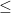1.

That was getting to be an algorithmic headache to implement, so I tried a simpler approach, as follows. First, run the LLSS method. If any reflectance values end up >1, constrain ALL of them to equal 1, and re-solve the optimization. This will usually cause some more values adjacent to the old contiguous regions to become >1, so constrain them in addition to the previous ones. Re-solve the optimization. Repeat the last two steps until all values are1. Here is an animation of this process, which I call the Iterative Least Log Slope Squared (ILLSS) process, applied to sRGB = (75, 255, 255):

To express the ILLSS algorithm mathematically, let’s begin with the LLSS optimization statement and add the additional equality constraints: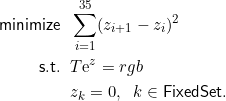“FixedSet” is the set of reflectance indices that are constrained to equal 1, or equivalently, the set of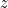indices constrained to equal zero (since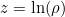). Initially, FixedSet is set to be the empty set. Each time the optimization is repeated, thevalues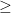0 have their indices added to this set.

We can define a matrix that summarizes the fixed set, for example: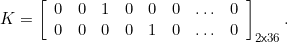This example indicates that there are two reflectance values being constrained (because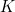has two rows), and the third and fifth reflectance values are the particular ones being constrained.

The Lagrangian formulation now has additional Lagrange multipliers, called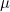, one for each of the constrained reflectances. The system of nonlinear equations produced by finding a stationary point of the Lagrangian (setting partial derivatives of the Lagrangian with respect to each set of variables (,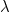, and) equal to zero) is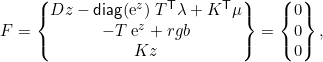where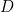is the same 36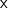36 tridiagonal matrix presented earlier. As before, we solve this nonlinear system with Newton’s method. Forming the Jacobian matrix,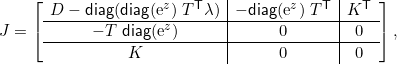the change in the variables with each Newton iteration is found by solving the linear system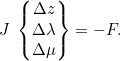Here is a Matlab program that performs the ILLSS (Iterative Least Log Slope Squared) optimization. I included a check for the two special cases of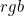= (0,0,0) or (255,255,255), which simply return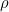= (0.0001, 0.0001, …, 0.0001) or (1, 1, …, 1). The additional special case of (255,255,255) is needed because numerical issues arise if thematrix grows to 3636, as it would in that second special case. This program works in Octave as well.

Optimization Packages
An alternative to the ILLSS method would be to use a complete nonlinear optimization package to solve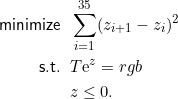I am aware of two commercial optimization packages that work. First, Excel has an Add-In called the Solver. I believe it comes with every version of Excel, but you may have to enable it to get it to appear in the Data tab/menu. One of its solving methods is called “GRG Nonlinear” and it works very well on this problem. Here is an Excel spreadsheet with the optimization set up. Just follow the instructions on the sheet to get a physically valid reflectance curve from a specified sRGB triplet.

The second optimization package I recommend is the Optimization Toolbox in Matlab. I don’t know whether or not the free alternative to Matlab (called Octave) has similar optimization capabilities. Here is a Matlab function that does the inequality-constrained optimization.

I consider both of these optimization packages to be “heavy artillery” for solving this relatively simple optimization problem, in comparison to the tight and efficient ILLSS solution method.